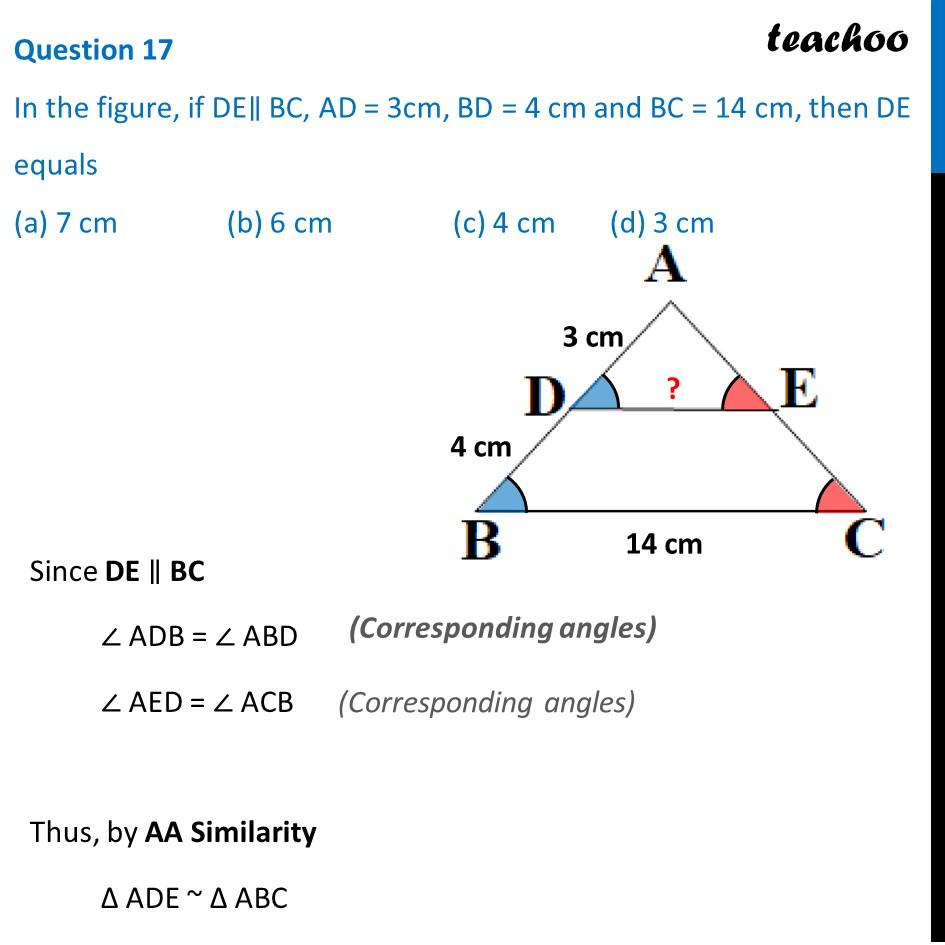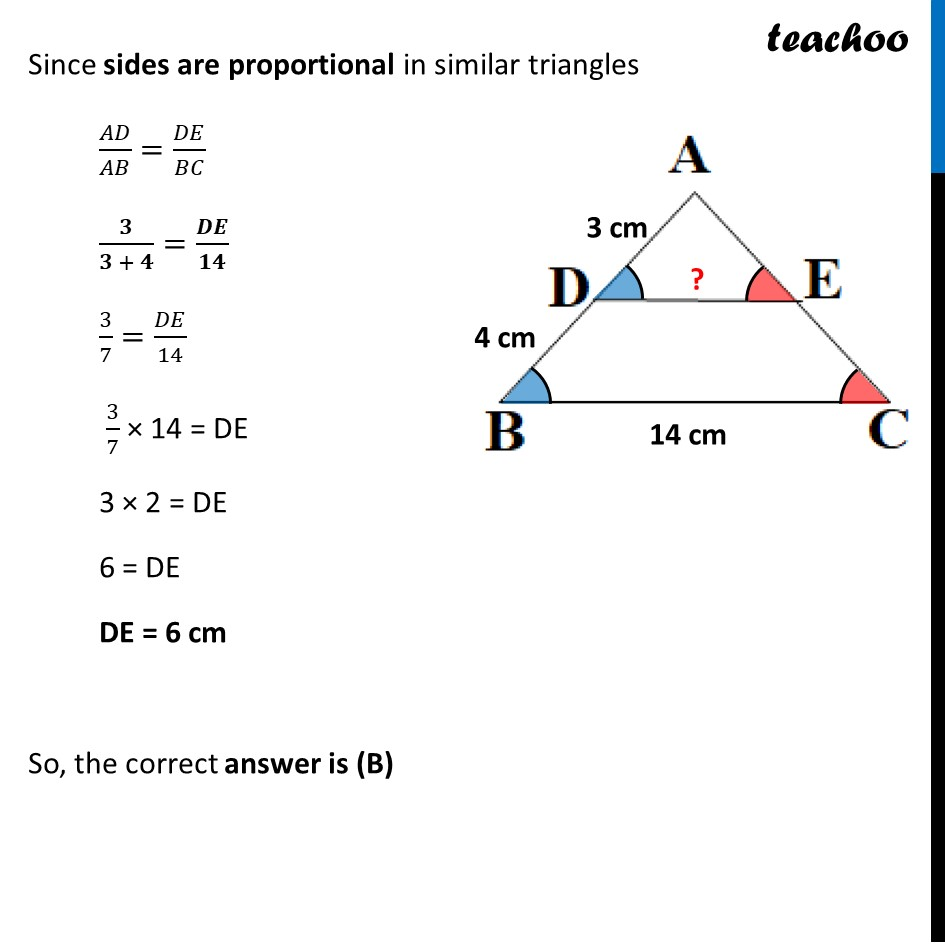## (a) 7 cm    (b) 6 cm     (c) 4 cm      (d) 3 cm

This question is inspired from Question 2 - NCERT Exemplar - MCQ - Chapter 6 Class 10 Triangles

##Advertisement (adsbygoogle = window.adsbygoogle || []).push({});Advertisement (adsbygoogle = window.adsbygoogle || []).push({});

1. Class 10
2. Solutions of Sample Papers for Class 10 Boards
3. CBSE Class 10 Sample Paper for 2022 Boards - Maths Standard [MCQ]

Transcript

Question 17 In the figure, if DE∥ BC, AD = 3cm, BD = 4 cm and BC = 14 cm, then DE equals (a) 7 cm (b) 6 cm (c) 4 cm (d) 3 cm Since DE ∥ BC ∠ ADB = ∠ ABD ∠ AED = ∠ ACB Thus, by AA Similarity Δ ADE ~ Δ ABC Since sides are proportional in similar triangles 𝐴𝐷/𝐴𝐵=𝐷𝐸/𝐵𝐶 𝟑/(𝟑 + 𝟒)=𝑫𝑬/𝟏𝟒 3/7=𝐷𝐸/14 3/7 × 14 = DE 3 × 2 = DE 6 = DE DE = 6 cm So, the correct answer is (B)